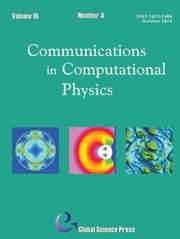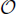Home
Hostname: page-component-544b6db54f-5rlvm Total loading time: 0.209 Render date: 2021-10-19T13:42:05.146Z Has data issue: true Feature Flags: { "shouldUseShareProductTool": true, "shouldUseHypothesis": true, "isUnsiloEnabled": true, "metricsAbstractViews": false, "figures": true, "newCiteModal": false, "newCitedByModal": true, "newEcommerce": true, "newUsageEvents": true }Communications in Computational Physics

# Exact Artificial Boundary Condition for the Poisson Equation in the Simulation of the 2D Schrödinger-Poisson System

Published online by Cambridge University Press:  03 June 2015

*
Corresponding author.Email:yong.zhang@univie.ac.at

## Abstract

We study the computation of ground states and time dependent solutions of the Schrödinger-Poisson system (SPS) on a bounded domain in 2D (i.e. in two space dimensions). On a disc-shaped domain, we derive exact artificial boundary conditions for the Poisson potential based on truncated Fourier series expansion in θ, and propose a second order finite difference scheme to solve the r-variable ODEs of the Fourier coefficients. The Poisson potential can be solved within(MNlogN) arithmetic operations where M,N are the number of grid points in r-direction and the Fourier bases. Combined with the Poisson solver, a backward Euler and a semi-implicit/leap-frog method are proposed to compute the ground state and dynamics respectively. Numerical results are shown to confirm the accuracy and efficiency. Also we make it clear that backward Euler sine pseudospectral (BESP) method in  can not be applied to 2D SPS simulation.

Type
Research Article
Information
Communications in Computational Physics , September 2014 , pp. 764 - 780

## Access options

Get access to the full version of this content by using one of the access options below. (Log in options will check for institutional or personal access. Content may require purchase if you do not have access.)

## References

Ando, T., Fowler, B. and Stern, F., Electronic properties of two-dimensional systems, Rev. Modern Phys., 54 (1982), 437672.CrossRefGoogle Scholar
Bastard, G., Wave Mechanics Applied to Semiconductor Heterostructure, Wiley, 1991.Google Scholar
Bao, W. Z. and Cai, Y. Y., Mathematical theory and numerical methods for Bose-Einstein condensation, Kinet. Relat. Models, 6 (2013), 1135.Google Scholar
Bao, W. Z. and Cai, Y. Y., Optimal error estimate of finite difference methods for the Gross-Pitavskii equation with angular momentum rotation, Math. Comp., 82 (2013), 99128.CrossRefGoogle Scholar
Bao, W. Z., Cai, Y. Y. and Wang, H. Q., Efficient numerical methods for computing ground states and dynamics of dipolar BoseEinstein condensates, J. Comput. Phys., 229 (2010), 78747892.CrossRefGoogle Scholar
Bao, W. Z., Chern, I-L. and Lim, F. Y., Efficient and spectrally accurate numerical methods for computing ground and first exrefd states in Bose-Einstein condensates, J. Comput. Phys., 219 (2006), 836854.CrossRefGoogle Scholar
Bao, W. Z. and Du, Q., Computing the ground state solution of Bose-Einstein condensates by a normalized gradient flow, SIAM J. Sci. Comput., 25 (5) (2004), 16741697.CrossRefGoogle Scholar
Bao, W. Z., Du, Q. and Zhang, Y. Z., Dynamics of rotating Bose-Einstein condensates and its efficient and accurate numerical computation, SIAM J. Appl. Math., 66 (3) (2006), 758786.CrossRefGoogle Scholar
Bao, W. Z. and Han, H. D., High-order local artifcial boundary conditions for problems in unbounded domains, Comput. Methods Appl. Mech. Engrg., 188 (2000), 455471.CrossRefGoogle Scholar
Bao, W. Z., Jian, H. Y., Norbert, N. J. and Zhang, Y., Dimension reduction of the Schrödinger equation with Coulomb and anisotropic confining potentials, SIAM J. Appl. Math., 73 (6) (2013), 21002123.CrossRefGoogle Scholar
Bao, W. Z., Jin, S. and Markowich, P. A., Time-splitting spectral approximations for the Schrödinger equation in the semiclassical regime, J. Comput. Phys., 175 (2002), 487524.CrossRefGoogle Scholar
Bao, W. Z., Mauser, N. J. and Stimming, H. P., Effective one particle quantum dynamics of electrons: A numerical study of the Schrödinger-Poisson-Xa model, Comm. Math. Sci., 1 (2003), 809831.CrossRefGoogle Scholar
Bardos, C., Erdős, L., Golse, F., Mauser, N. J. and Yau, H.-T., Derivation of the Schrödinger-Poisson equation from the quantum N-particle Coulomb problem, C. R. Math. Acad. Sci. Paris, 334(6) (2002), 515520.CrossRefGoogle Scholar
Bardos, C., Golse, F. and Mauser, N. J., Weak coupling limit of the N-particle Schrödinger equation, Methods Appl. Anal., 7(2) (2000), 275293.Google Scholar
Abdallah, N. Ben, Castella, F. and Méhats, F., Time averaging for the strongly confined non-linear Schrödinger equation, using almost-periodicity, J. Differential Equations, 245 (2008), 154200.CrossRefGoogle Scholar
Chen, H., Su, Y. and Shizgal, B. D., A direct spectral collocation Poisson solver in polar and cylindrical coordinates, J. Comput. Phys., 160 (2000), 453469.CrossRefGoogle Scholar
Dong, X. C., A short note on simplified pseudospectral methods for computing ground state and dynamics of spherically symmetric Schrödinger-Poisson-Slater system, J. Comput. Phys., 230 (2011), 79177922.CrossRefGoogle Scholar
Erdős, L. and Yau, H.-T., Derivation of the nonlinear Schrödinger equation from a many body Coulomb system, Adv. Theor. Math. Phys., 5 (2001), 11691205.CrossRefGoogle Scholar
Ethridge, F. and Greengard, L., A new fast-multipole accelerated Poisson solver in two di-mensions, SIAM J. Sci. Comput., 23 (3) (2001), 741760.CrossRefGoogle Scholar
Ferry, D. K. and Goodnick, S. M., Transport in Nanostructures, Cambridge University Press, Cambridge, UK, 1997.CrossRefGoogle Scholar
Fornberg, B., A pseudospectral approach for polar and spherical geometries, SIAM J. Sci. Comput., 16 (5) (1995), 10711081.CrossRefGoogle Scholar
Genovese, L., Deutsch, T., Neelov, A., Goedecker, S. and Beylkin, G., Efficient solution of Poisson equation with free boundary conditions, J. Comput. Chem., 125 074105 (2006).Google Scholar
Greengard, L. and Rokhlin, V., A new version of the fast multipole method for the Laplace equation in three dimensions, Acta Numerica, 6 (1997), 229269.CrossRefGoogle Scholar
Han, H. D. and Bao, W. Z., Error estimates for the finite element approximation of problems in unbounded domains, SIAM J. Numer. Anal.,47(4) (2000), 11011119.CrossRefGoogle Scholar
Han, H. D. and Huang, Z. Y., Exact artificial boundary conditions for the Schrödinger equation in R2, Commun. Math. Sci., 2 (1) (2004), 7994.CrossRefGoogle Scholar
Harrison, R., Moroz, I. M. and Tod, K. P., A numerical study of Schrödinger-Newton equations, Nonlinearity, 16 (2003), 101122.CrossRefGoogle Scholar
Lai, M.-C., Lin, W.-W. and Wang, W., A fast spectral/difference method without pole conditions for Poisson-type equations in cylindrical and spherical geometries, IMA J. Numer. Anal., 22 (2002), 537548.CrossRefGoogle Scholar
Lai, M.-C. and Wang, W.-C., Fast direct solvers for Poisson equation on 2D polar and spherical geometries, Numer. Methods Partial Differential Equations, 18 (2002), 5668.CrossRefGoogle Scholar
Shen, J., Efficient spectral-Galerkin methods III: Polar and cylindrical geometries, SIAM J. Sci. Comput., 18 (6) (1997), 15831604.CrossRefGoogle Scholar
Soba, A., A finite element method solver for time-dependent and stationary Schrödinger equations with a generic potential, Commun. Comput. Phys., 5 (2009), 914927.Google Scholar
Tan, I. H., Snider, G. L., Chang, L. D. and Hu, E. L., A self-consistent solution of Schrödinger- Poisson equations using a nonuniform mesh, J. Appl. Phys., 68 (1990), 40714076.CrossRefGoogle Scholar
Zhang, Y., Optimal error estimates of compact finite difference discretizations for the Schrödinger-Poisson system, Commun. Comput. Phys., 13 (2013), 13571388.CrossRefGoogle Scholar
Zhang, Y. and Dong, X. C., On the computation of ground state and dynamics of Schrödinger-Poisson-Slater system, J. Comput. Phys., 230 (2011), 26602676.CrossRefGoogle Scholar
7
Cited by

# Send article to Kindle

Note you can select to send to either the @free.kindle.com or @kindle.com variations. ‘@free.kindle.com’ emails are free but can only be sent to your device when it is connected to wi-fi. ‘@kindle.com’ emails can be delivered even when you are not connected to wi-fi, but note that service fees apply.

Find out more about the Kindle Personal Document Service.

Exact Artificial Boundary Condition for the Poisson Equation in the Simulation of the 2D Schrödinger-Poisson System
Available formats
×

# Send article to Dropbox

To send this article to your Dropbox account, please select one or more formats and confirm that you agree to abide by our usage policies. If this is the first time you use this feature, you will be asked to authorise Cambridge Core to connect with your <service> account. Find out more about sending content to Dropbox.

Exact Artificial Boundary Condition for the Poisson Equation in the Simulation of the 2D Schrödinger-Poisson System
Available formats
×

# Send article to Google Drive

To send this article to your Google Drive account, please select one or more formats and confirm that you agree to abide by our usage policies. If this is the first time you use this feature, you will be asked to authorise Cambridge Core to connect with your <service> account. Find out more about sending content to Google Drive.

Exact Artificial Boundary Condition for the Poisson Equation in the Simulation of the 2D Schrödinger-Poisson System
Available formats
×
×